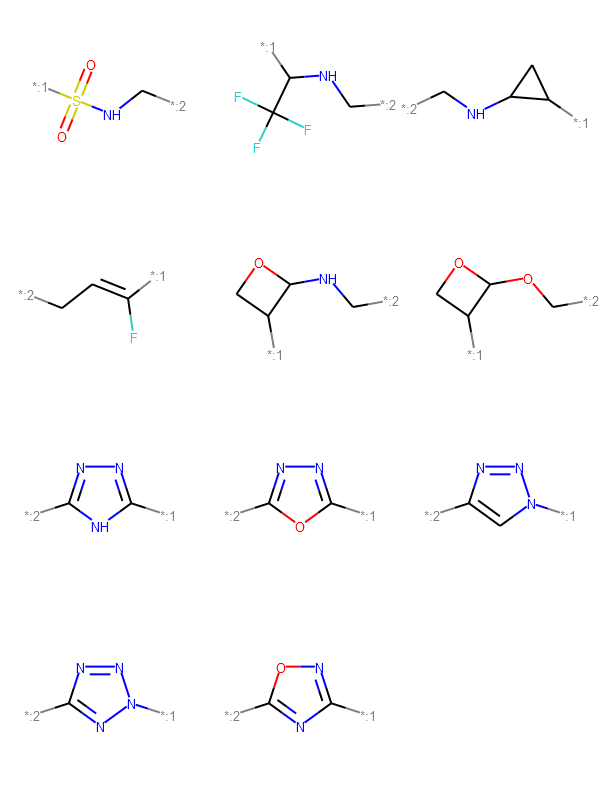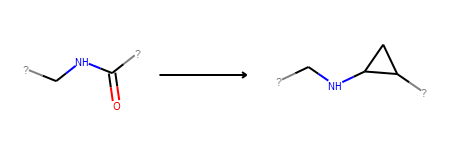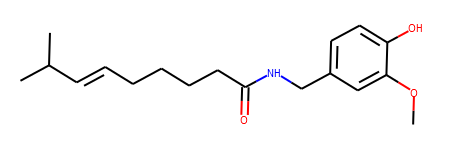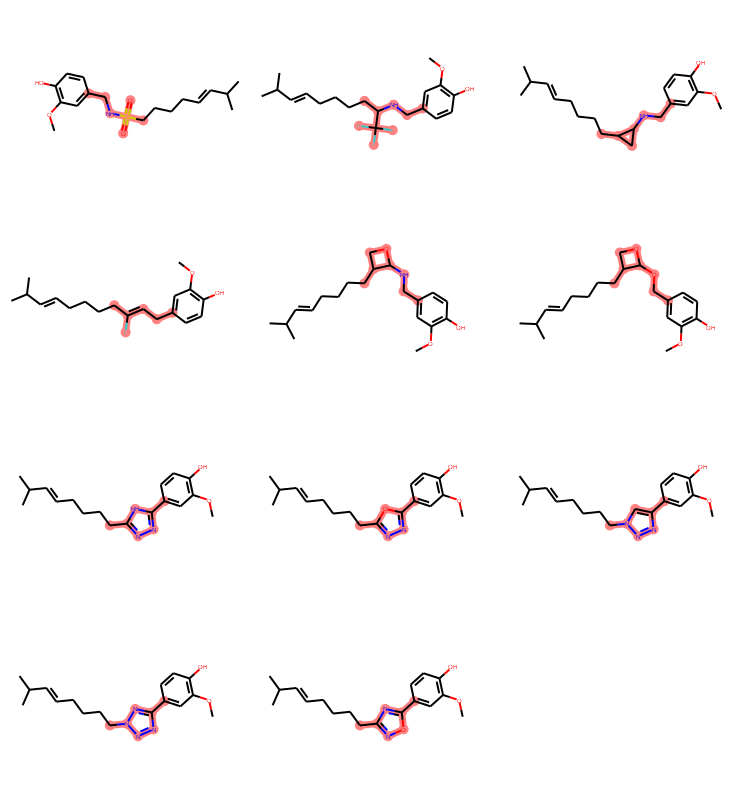## Wednesday, February 7, 2018

### Simple isostere replacement

This post is inspired by a question that came up on the mailing list recently. The poster was looking for help doing bioisotere replacements with the RDKit. The topic is interesting and seems useful enough to merit a short blog post.
In :
```# start with the usual imports
from rdkit import Chem
from rdkit.Chem import AllChem, Draw
from rdkit.Chem.Draw import IPythonConsole
import time
from rdkit import rdBase
print(time.asctime())
print(rdBase.rdkitVersion)
```
```Wed Feb  7 15:10:15 2018
2017.09.3
```
In :
```isostere_smis = ('[*:1]S(=O)(=O)NC[*:2]',
'[*:1]C(C(F)(F)(F))NC[*:2]',
'[*:1]C1CC1NC[*:2]',
'[*:1]C(F)=CC[*:2]',
'[*:1]C1COC1NC[*:2]',
'[*:1]C1COC1OC[*:2]',
'[*:1]C1=NN=C([*:2])N1',
'[*:1]C1=NN=C([*:2])O1',
'[*:1]N1N=NC([*:2])=C1',
'[*:1]N1N=NC([*:2])=N1',
'[*:1]C1=NOC([*:2])=N1')
isosteres = [Chem.MolFromSmiles(x) for x in isostere_smis]
Draw.MolsToGridImage(isosteres)
```
Out:And now we'll use those to build the reactions that we'll use to apply the bioisosteric transformations.
In :
```def buildIsostereReaction(start,replacement):
qps.aromatizeIfPossible = False
qps.makeAtomsGeneric = False
qps.makeBondsGeneric = False
qps.makeDummiesQueries = True
product = AllChem.ChemicalReaction()
product.Initialize()
return product
```
Create all the reactions and take a look at one of them to get a sense of what we're doing:
In :
```amide = Chem.MolFromSmiles('[*:1]C(=O)NC[*:2]')
isostereReactions =[buildIsostereReaction(amide,x) for x in isosteres]
isostereReactions
```
Out:Here are the functions for using those reactions:
In :
```def doIsostereReplacement(mol,rxn):
ps = rxn.RunReactants((mol,))
res = [x for x in ps]
return res
def doIsostereReplacements(mol,rxns):
res = []
for i,rxn in enumerate(rxns):
seenSoFar=set()
ims = doIsostereReplacement(mol,rxn)
p0 = rxn.GetProductTemplate(0)
# save where the match is
for im in ims:
smi = Chem.MolToSmiles(im,True)
if smi not in seenSoFar:
im.coreMatches = im.GetSubstructMatch(p0)
res.append(im)
return res
```
We'll use capsaicin as our example
In :
```mol =Chem.MolFromSmiles('COc1cc(CNC(=O)CCCC/C=C/C(C)C)ccc1O')
mol
```
Out:Get all the products of applying our isosteric replacements:
In :
```subs = doIsostereReplacements(mol,isostereReactions)
len(subs)
```
Out:
`11`
Let's look at what we got back and, though it's mostly obvious, highlight the replacement:
In :
```atomsToHighlight = [sub.coreMatches for sub in subs]
Draw.MolsToGridImage(subs,subImgSize=(250,200),highlightAtomLists=atomsToHighlight)
```
Out:As I promised above, this is a short one, so that's it for now.
Comments, questions, or pointers to other lists of useful bioisosteres are welcome!Anonymous said...

Dear Greg,

Thank you for your useful blog post!
I have a question. Bioisosteric replacement is similar to MMP replacement. In rdkit Contrib/mmpa provides mol_tranform.py script. The script can transform query molecules to new molecules with given Matched Pair Rules. In mol_transform.py, AdjustQueryParameters class is not used.
Could you please explain more details about the class if you have time?

Kind regards,
Takayuki

greg landrum said...

Hi,

-gregAnonymous said...

Hi Greg,